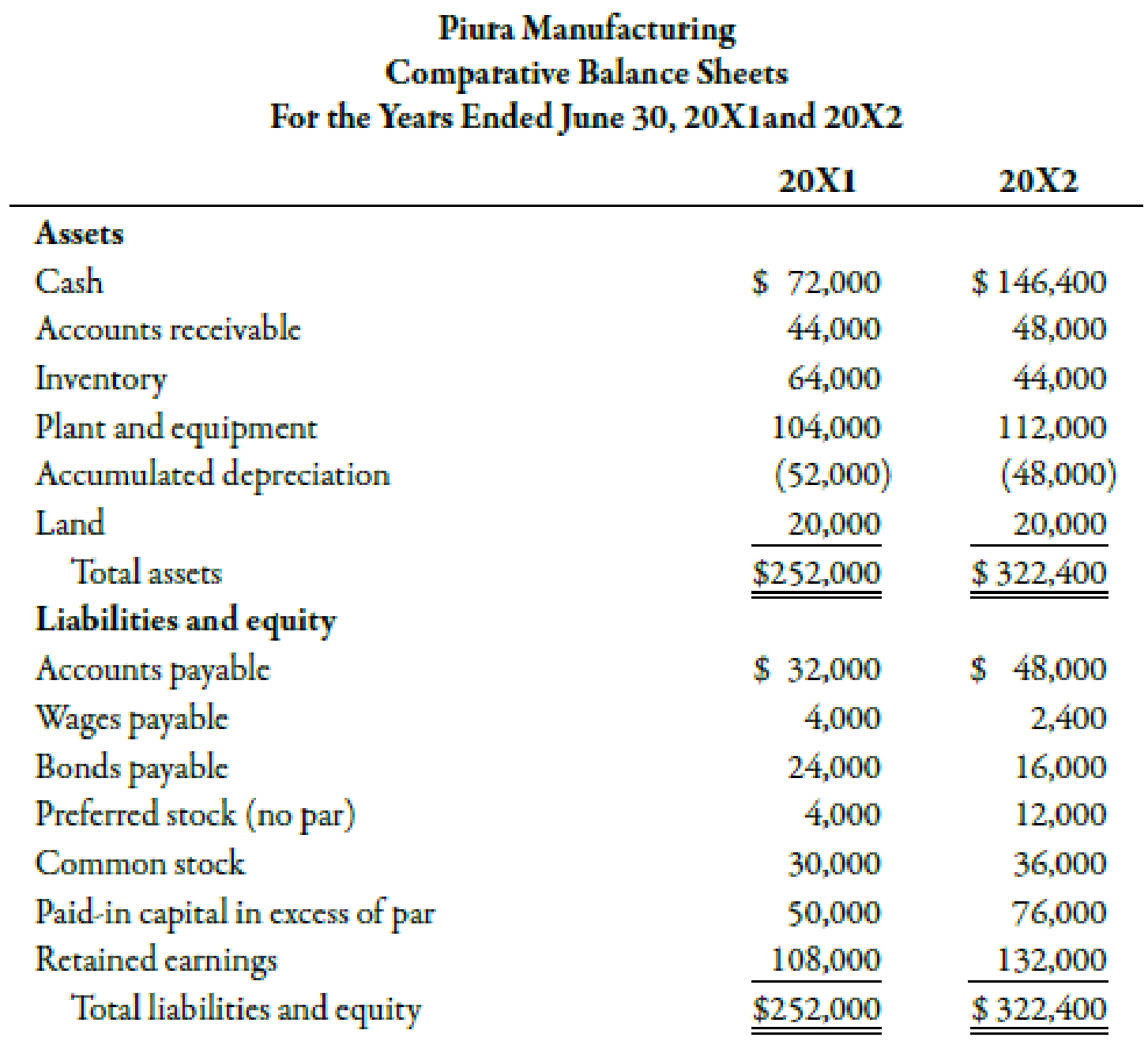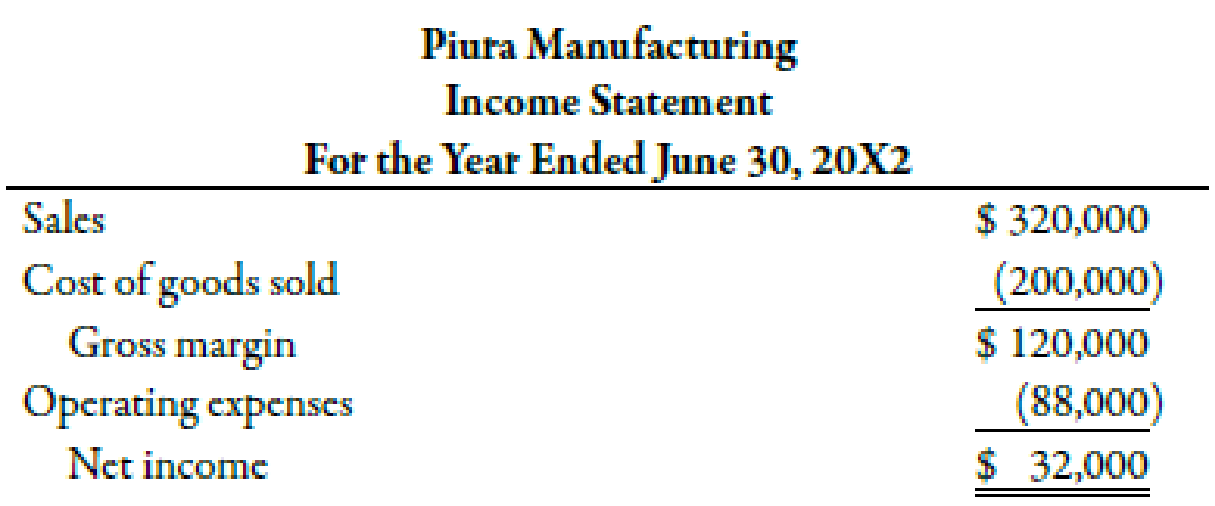Chapter 14, Problem 58C### Managerial Accounting: The Corners...

7th Edition
Maryanne M. Mowen + 2 others
ISBN: 9781337115773

#### Solutions

Chapter
Section### Managerial Accounting: The Corners...

7th Edition
Maryanne M. Mowen + 2 others
ISBN: 9781337115773
Textbook Problem
581 views

# The comparative balance sheets and income statement of Piura Manufacturing follow.Additional transactions for 20X2 were as follows: a. Cash dividends of $8,000 were paid. b. Equipment was acquired by issuing common stock with a par value of$6,000. The fair market value of the equipment is $32,000. c. Equipment with a book value of$12,000 was sold for $6,000. The original cost of the equipment was$24,000. The loss is included in operating expenses. d. Two thousand shares of preferred stock were sold for $4 per share. Required: 1. Prepare a schedule of operating cash flows using (a) the indirect method and (b) the direct method. 2. Prepare a statement of cash flows using the indirect method. 3. Prepare a statement of cash flows using a worksheet similar to the one shown in Example 14.8 (p. 804). 4. Form a group with two to four other students, and discuss the merits of the direct and indirect methods. Which do you think investors might prefer? Should the FASB require all companies to use the direct method? 1. (a). To determine Construct a schedule of operating cash flows by indirect method. Explanation Cash Flows from Operating Activities: This category of a cash flow statement shows the operational and profit generating activities in a firm. The operating cash flows increase or decrease the current assets and current liabilities of a firm. The schedule for operating cash flows using indirect method is shown in the table below:  PM Company Schedule for Operating Cash Flows For the year ended June 30, 20X2 Operating Cash Flows: Particulars Amount ($) Net income 32,000 Add/ Less: Increase in accounts receivable1 (4,000) Decrease in inventories2 20,000 Increase in accounts payable3 16,000 Decrease in wages payable4 (1,600) Loss on sale of equipment5 6,000 Depreciation expense6 8,000 Net cash from operating activities 76,400

Table (1)

Therefore, the net cash from operating activities is $76,400. Working Note: 1. Calculation of difference in accounts receivable: (DifferenceinAccountsReceivable)=(AmountofAccountsReceivableof20X2AmountofAccountsReceivableof20X1)=($48,000$44,000)=$4,000

2.

Calculation of difference in amount of inventories:

(DifferenceinAmountofInventories)=(AmountofInventoriesof20X2AmountofInventoriesof20X1)=($44,000$64,000)=$20,000 3. Calculation of difference in accounts payable: (DifferenceinAccountsPayable)=(AmountofAccountsPayableof20X2AmountofAccountsPayableof20X1)=($48,000$32,000)=$16,000

4

1.

(b).

To determine

Construct a schedule of operating cash flows by direct method.

2.

To determine

Construct a statement of cash flows using the indirect method.

3.

To determine

Construct a statement of cash flows with the help of a worksheet.

4.

To determine

Explain the merits and demerits of the direct and indirect method. Also, explain the method preferably to be used by the investors. Explain whether it is mandatory by FASB for the companies to use direct method.

### Still sussing out bartleby?

Check out a sample textbook solution.

See a sample solution

#### The Solution to Your Study Problems

Bartleby provides explanations to thousands of textbook problems written by our experts, many with advanced degrees!

Get Started

#### Find more solutions based on key concepts